[See Low Graphics Version]

## 00-Level Mathematics Courses at Trent University (2004-2005)

Notes:

 100-Level ....... 200-Level ....... 300-Level ....... 400-Level## 100-Series CoursesMath. 105H Applied Calculus Fall 2004 and Winter 2005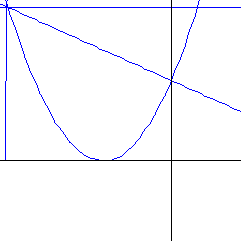Approximating a tangent line with a convergent sequence of secants. An introduction to the methods and applications of calculus. Derivatives, exponential and logarithmic functions, optimization problems, related rates, integration, partial derivatives, differential equations. Selected applications from the natural and social sciences. Not available to students enrolled in or with credit for Mathematics 110. Not for credit towards a major in Mathematics. Prerequisite: A Grade 12U or U/C mathematics course or an OAC mathematics course. Meetings: Three hour lecture and one hour workshop weekly. Timetables: Instructors: Fall section: Xiaorang Li Winter section: Xiaorang Li Oshawa section: Fred Pulfer

Math. 110 Calculus of one variable Fall-Winter 2004-2005
 An examination of the concepts and techniques of calculus, with applications to other areas of mathematics and the physical and social sciences. Prerequisite: Grade 12 Advanced Functions and Introductory Calculus or Grade 13/OAC calculus - with at least 60% or equivalent in each case. Meetings: Three lectures and one seminar weekly. Timetables: Instructors: Section 110A   Stefan Bilaniuk Section 110B   Ion Rada For more information, please see the MATH 110 home page.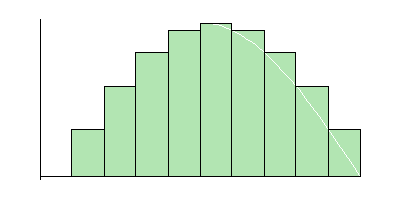Computing an integral with a convergent sequence of Riemann sums.

Math. 135H Linear Algebra I: Matrix Algebra Fall 2004 and Winter 2004-2005

Vectors, systems of linear equations, matrices, determinants, linear transformations, eigenvalues and eigenvectors. Excludes Mathematics 130.

• Prerequisite: A Grade 12U or U/C mathematics course or OAC Mathematics credit with at least 60%.
• Meetings: Three hours lecture and one hour workshop weekly.
• Timetables:
• Instructors:

Math. 150 A non-calculus-based introduction to probability & statistical methods Fall-Winter 2004-2005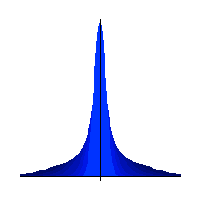Seven Gaussian distributions of increasing variance. Data summary, elementary probability, estimation, hypothesis testing, comparative methods, analysis of variance, regression, nonparametric methods, introduction to elementary applications of statistical computing. This course uses highschool mathematics as a foundation and involves the use of computers.Not credited toward Mathematics major requirements, nor available to students enrolled in, or with credit for Mathematics-Statistics 251H or Mathematics 110. Meetings: Three lectures weekly, one-hour problems session fortnightly. Class timetable Instructor: Michelle Boue. For more information, please see the Math 150 Homepage or the Statistics Courses Page.

Math. 155H Introduction to probability Fall2004 and Winter 2005

Probability, random variables, probability distributions. Not available to students with credit for Mathematics-Statistics 251H.## 200-Series CoursesMath. 200 Calculus in several dimensions Fall-Winter 2004-2005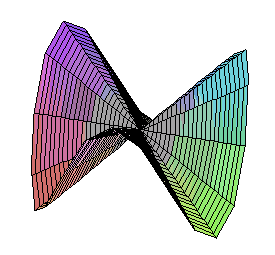The Monkey's Saddle is not differentiable at zero, but all tangent lines exist there. Vector geometry, curves, surfaces in three dimensions. Partial differentiation and applications, multiple integrals. Vector calculus. Prerequisite: Mathematics 110. Class timetable Instructor:   Wendi Morrison

Math.-Physics 205H Ordinary Differential Equations Fall 2004
 Prerequisite: Mathematics 110. Corequisite: Mathematics 200. Recommended: Mathematics 130 or 135H. Meetings: Three lectures and one tutorial weekly. Class timetable Instructor: Xiaorang Li Synopsis::  First order equations; qualitative and numerical methods. Second order linear equations. Applications to physical and biological models. Laplace transforms. Power series solutions.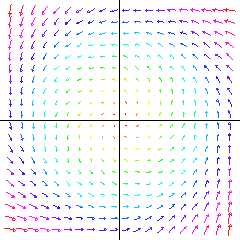A two-dimensional vector field determines a flow in the plane.

Overview: Ordinary differential equations (ODEs) model physical systems which evolve continuously in time. For example, suppose the state of the system is described by a single variable $x\left(t\right)$, and satisfies the equation:
 d x(t) ----- = -x(t). d t
If $x\left(0\right)=5$, then the unique solution to this equation is the curve $x\left(t\right) = 5 e-t$. This curve starts at $5$, and asymptotically approaches $0$. We say that $0$ is an equilibrium state for the system.

Suppose the state of the system at time $t$ is given by a real vector x(t) in RN. Then an ODE for this system has the form:
 d x ----- = V[x(t)]. d t
This says that the velocity of the system (namely $d$x/dt) is a determined entirely by it's state (namely x), via some function $V:$RN ---->RN.

`     `

ODEs are ubiquitous in applied mathematics. For example:

• In physics: ODEs model trajectories in classical and relativistic mechanics.
• In chemistry: ODEs describe the reaction kinetics of chemical systems.
• In biology: ODEs describe the evolving populations of interacting species, fluctuating endocrine levels in the body, or neural activity in the brain.
• In economics: ODEs describe business cycles.

Given an ODE, we can ask four questions:

1. Do solutions exist? In other words, given an initial state x0, is there a smooth curve x(t) satisfying x(0)=x0 and the ODE?
2. Is this solution unique?
3. What is an explicit formula describing the solution?
4. What is the long-term qualitative behaviour of the system?

Math. 206H Analysis I: Introduction to Analysis Fall 2004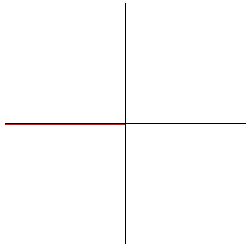A sequence of successively higher order Taylor polynomials converge to the sine function. The real number system. Limits. Continuity. Differentiability. Mean-value theorem. Convergence of sequences and series. Uniform convergence. Prerequisite: Mathematics 110. Corequisite: Mathematics 200. Meetings: Three hours weekly. Class timetable Instructor: Xiaorang Li

Math. 207H Introduction to numerical & computational methods Winter 2005

Error analysis, non-linear equations, linear systems, interpolation methods, numerical differentiation and integration and initial value problems.

Math. 226H Geometry I: Euclidean geometry Fall 2004

Elements of Euclidean geometry stressing links to modern mathematical methods. Geometric transformations and symmetry. Recommended for Education students.

• Prerequisite: Either Mathematics 105H or 110 (with OAC Algebra/Geometry recommended), or 135H.
• Meetings: Two lectures and one tutorial weekly.
• Class timetable
• Instructor:  Reem Yassawi

Math. 235H Linear Algebra II: Vector Spaces Winter 2005

Vector spaces, basis and dimension, inner product spaces, orthogonality, linear transformations, diagonalization, determinants, eigenvalues, quadratic forms, least squares, the singular value decomposition. Excludes Mathematics 130.

• Prerequisite: Mathematics 135H.
• Meetings: Three hours lecture and one hour tutorial weekly.
• Class timetable
• Instructor:   David Poole

Math.-Comp. Sci. 260 Discrete Structures Fall-Winter 2004-2005

Mathematics related to computer science including propositional logic, recursive functions, combinatorics, graphs and networks, Boolean algebras. Applications to languages, analysis of algorithms, optimization problems, coding theory, and circuit design.

• Prerequisite: Mathematics 110 or 130 or 135H; or Computer Science 102H together with one Grade 12 U or U/C or OAC credit in Mathematics.
• Recommended: OAC Finite Mathematics.
• Meetings: Three hours weekly.
• Class timetable
• Instructor:   Marco Pollanen

Math. 280 Mathematics for the Contemporary Classroom Fall-Winter 2004-2005

A course in mathematics and mathematical thinking for prospective school teachers. Number systems and counting, graphs and networks, probability and statistics, measurement and growth, symmetry, computers and mathematics. Not available to students enrolled in or with credit for any of Mathematics 110, Mathematics 135H or Mathematics-Computer Science 260 or their equivalents. Not for credit towards any major in Mathematics.## 300-Series CoursesMath.-Physics 305H Partial Differential Equations Fall 2004
 Prerequisite: Mathematics 205H. Corequisite: Mathematics 200. Meetings: Three hours weekly. Class timetable Instructor: Marcus Pivato For more information, please see the MATH 305 home page. Synopsis: We'll focus on the following topics: The Heat equation, Wave equation, Laplace equation, and Poisson equation in one, two, and three dimensions, in Cartesian, Polar, and Spherical coordinates. Solution methods using eigenfunction expansions (Fourier series, Fourier transforms, Bessel functions) Solution methods using convolutional transforms (Gauss-Weierstrass kernel, d'Alembert method).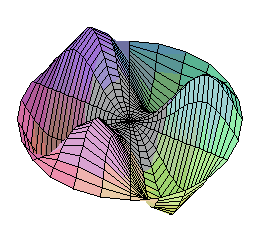The Wave Equation: A vibrational mode of a circular membrane.

Overview: Partial differential equations (PDEs) model physical systems which evolve continuously in time, and whose physical state is described by some continuous function in space. For example, suppose we pour some ink into a flat tray of water. Let $p\left(x,y;t\right)$ describe the concentration of ink in the tray at spatial coordinates $\left(x,y\right)$ and time $t$. Then the ink obeys the Heat Equation:
 d p d2 p d2 p ------ = ------ + ------ d t d x2 d y2
This equation says the ink will diffuse from regions of high concentration to regions of low concentration until it is uniformly distributed throughout the pan.

`     `

PDEs are ubiquitous in applied mathematics. For example:

• In physics: The Schrodinger equation describes the evolution of a quantum wavefunction. The Einstein equation describes the curvature of space-time.
• In chemistry: Reaction-diffusion equations describe spatially distributed chemical systems.
• In biology: PDEs describe ontogenic processes and ecosystems.

Given a PDE we can ask four questions:

1. Do solutions exist?
2. Is the solution unique?
3. What is an explicit formula describing the solution?
4. What is the long-term qualitative behaviour of the system?

Math. 306H Analysis II: Complex Analysis Winter 2005Colour-coding the complex plane in polar coordinates Functions of a complex variable, analytic functions, complex integrals, Cauchy integral theorems, Taylor series, Laurent series, residue calculus. Corequisite: Mathematics 200. Meetings: Two lectures and one tutorial weekly. Class timetable Instructor: Marcus Pivato For more information, please see the MATH 306 home page.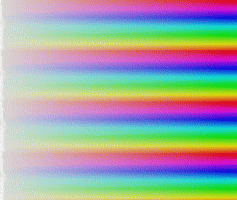The complex exponential map, seen through this colour-coding.

Math.-Physics 308H Methods of applied mathematics Not offered in 2004-2005

Differential equations in applied mathematics, including Bessel, Legendre, hypergeometric, Laguerre, Hermite, Chebyshev, etc. Series and numerical solutions. Properties of the special functions arising from these equations.

• Prerequisite: Mathematics-Physics 205H.
• Meetings: Three lectures and one tutorial weekly.
• Instructor: Not offered

Math. 310H Topology I: Metric spaces Winter 2005
 Limits and continuity. Completeness, compactness, the Heine-Borel theorem. Connectedness. Prerequisite: Mathematics 206H. Meetings: Two lectures and one tutorial weekly. Class timetable Instructor:   Ion Rada For more information, please see the MATH 310 home page.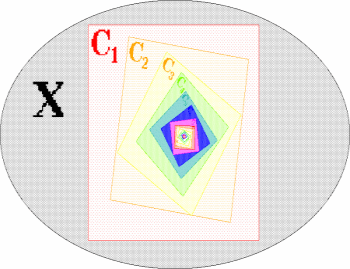The Chinese Box Theorem says: if $A$1 > A2 > A3 > .... is a descending sequence of compact sets, then their common intersection is nonempty. This implies that every contraction mapping on a compact space has a fixed point.

Math.-Physics 311H Advanced classical mechanics Winter 2005

Applied mathematics as found in the classical mechanics of particles, rigid bodies and continuous media. Motion of rigid bodies, Lagrangian mechanics, Hamiltonian mechanics, dynamics of oscillating systems.

Math.-Physics 312H Classical mechanics Fall 2004

Applied mathematics as found in the classical mechanics of particles. One-dimensional motion, vector differential operators, three-dimensional motion, moving and rotating coordinate systems, central forces, systems of particles.

• Prerequisites: Mathematics 110 and Physics 100 or permission of the instructor.
• Pre or co-orequisite: Mathematics 200.
• Meetings: Three lectures and tutorial weekly.
• Class timetable
• Taught by the Department of Physics.

Math. 322 Number Theory Fall-Winter 2004-2005
 Prerequisites: Mathematics 110, and 130 or 235H. Meetings: Three hours weekly. Class timetable Instructor:   Ion Rada Overview: Number theory is one of the oldest and richest areas of mathematics, and ubiquitous in contemporary mathematical research. We will likely examine the following topics: Prime Numbers: The Fundamental Theorem of Arithmetic says every number has a unique factorization into primes. We'll prove this theorem, and study its consequences. How many primes are there? Euclid proved there are an infinite number. How `densely' are the primes distributed in the natural numbers? Let P(n) be the number of primes less than $n$. For example, P(25)=9, because the primes less than 24 are $\left\{2,3,5,7,11,13,17,19,23\right\}$ The Prime Number Theorem states: $lim$n->oo   P(n) log(n) / n   =    1. This says that P(1 000 000)   =~    1 000 000/log(1 000 000)   =   (1 000 000)/(6 log{10)   =~    72 382. In other words, approximately 7.2% of the numbers less than 1 000 000 are prime. Are there patterns in prime numbers? Are there formulas for generating them? Is there an efficient way to test whether a given number is prime? RSA encryption uses prime factorization to create a public-key cryptosystem. An efficient factorization algorithm would break the encryption. We'll discuss this. Diophantine Equations: A Pythagorean triple is a triple of integers $\left(a,b,c\right)$ so that $a2+b2=c2$.For example: $32+ 42= 52$. Such numbers are called Pythagorean because they form the sides of a right-angle triangle. Such triples are quite hard to construct. ` `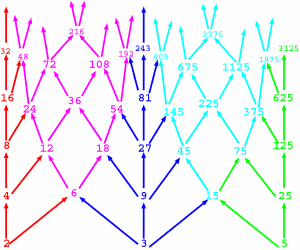The Lattice of Divisibility of integers. The equation $a2+b2=c2$ (with the stipulation that $a,b,c$ be integers) is an example of a Diophantine Equation. Such equations are very hard to solve. Another famous Diophantine equation is the Fermat Equation: . Fermat's famous Last Theorem says this equation has no nontrivial solutions for $n > 2$. Modular arithmetic is the arithmetic of 12 hour clocks, 7 day weeks, etc., and is fundamental to the theory of groups and rings. We will develop the basic theory of congruence relations. We will then look into congruence equations, focusing on such topics as: Fermat's Little Theorem and Wilson's Theorem. The Chinese Remainder Theorem, which solves systems of linear congruence equations. Quadratic congruences and the Quadratic Reciprocity theorem. Lucas' theorem, which describes the binomial ceofficients, mod $p$, and has applications to cellular automata.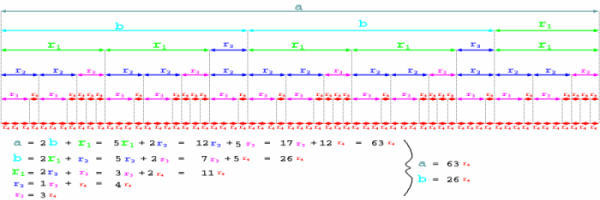The Euclidean algorithm is a method to compute the greatest common divisor of two numbers.

Math. 326H Geometry II: Projective & non-Euclidean geometries Not offered in 2004-2005
 Elements of projective and non-Euclidean geometries, including an introduction to axiomatic systems. Prerequisite: Mathematics 135H or permission of the instructor. Meetings: Two lectures and one tutorial weekly. Offered in alternate years but not in 2004-2005.

Math. 330 Algebra III: Groups, rings & fields Fall-Winter 2004-2005
 Prerequisite: Mathematics 235H. Meetings: Two lectures and one tutorial weekly. Class timetable Instructor: Marcus Pivato For more information, please see the MATH 330 home page. Overview: We will study three kinds of algebraic structures: groups, rings, and fields. Group Theory: Groups encode the symmetries other objects. For example: Dihedral groups describe the symmetries of figures in the plane. Polyhedral groups describe the symmetries of polyhedra in three dimensions. Tiling groups describe the symmetries of infinite tilings of the plane. Linear Groups describe symmetries of objects in Euclidean space of many dimensions. Lie Groups describe the symmetries of curves, surfaces, and other manifolds. Groups can also be thought of as `abstract spaces', or used to encode geometric/topological information. For example Vector spaces are groups with an explicitly spatial structure. Homotopy groups describe how curves can continously deform in a surface or other space. They encode information about the topology of the space. Holonomy groups describe the distortions introduced by traveling through curved space. Ring Theory: Rings are algebraic structures which encode abstract arithmetic. Number rings extend the arithmetic of integers. Many problems in number theory (eg. Fermat's Last Theorem) can be better understood by contextualizing them within ring theory. Coordinate rings describe the geometry of a curve, surface, or other space. Many geometric questions about the space can be translated into algebraic questions about the ring, and answered using algebraic methods. Operator Algebras: are rings of matrices acting on a vector space. They arises in areas from dynamical systems to quantum theory. Field Theory: A field is a special kind of ring with a particularly rich algebraic structure. The rational numbers, the real numbers, and the complex numbers are examples of fields. Field theory reveals important limitations to mathematical methods. For example: You can't trisect an angle or construct a 7-gon using a compass and straightedge. It's not that we haven't figured it out yet; you simply can't. You can solve any quadratic equation $ax2+ bx + c=0$ with the Quadratic Formula. However, there is no analogous quintic formula for solving a quintic equation $ax5+ bx4+ cx3+ dx2+ ex + f=0$. It's not that we haven't found it yet; there simply isn't one. ` `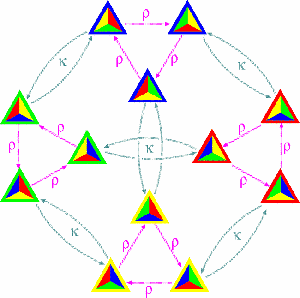The group of symmetries of a tetrahedron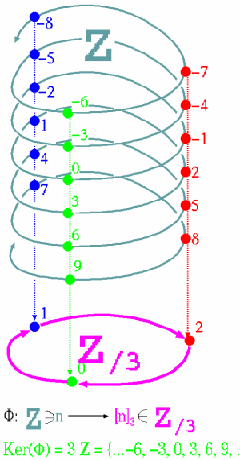A group epimorphism from Z into Z/3

Math.-Comp. Sci. 341 Linear and discrete optimization Fall-Winter 2004-2005

Introduction to the concepts, techniques and applications of linear programming and discrete optimization. Topics include the simplex method, dynamic programming, duality, game theory, transportation problems, assignment problems, matchings in graphs, network flow theory, and combinatorial optimization with emphasis on integer programming.

Math. 355 An introduction to statistical analysis Fall-Winter 2004-2005

Introduction to mathematical statistics: exploring and describing relationships, sampling, point and interval estimation, likelihood methods, hypothesis testing, comparative inferences, contingency tables, linear regression and correlation introductory multiple regression, design and analysis of experiments, nonparametric methods. Assumes a background in probability and uses introductory linear algebra. Excludes Mathematics-Statistics 252H.

Math.-Science 380 History of mathematics Not offered in 2004-2005

A study of the major currents of mathematical thought from ancient to modern times.

• Prerequisites: Mathematics 110 and 235H, or Mathematics 110 and permission of the instructor. Second-year students wishing to take the course must have permission of the instructor.
• Meetings: Three hours weekly.
• Instructor: Not offered

Math. 390 Reading-seminar course (Full) Fall and Winter (reading course)
Details may be obtained by consulting the Department of Mathematics.

Math. 391H Reading-seminar course (Half) Fall or Winter (reading course)
Details may be obtained by consulting the Department of Mathematics.## 400-Series CoursesMath. 406H Analysis III: Measure & integration Not offered in 2004-2005

Riemann and Lebesque measure, integration.

• Prerequisites: Mathematics 206H, 310H.
• Meetings: Three hours weekly.
• Instructor: Not offered

Math. 407H Analysis IV: Topics in analysis Not offered in 2004-2005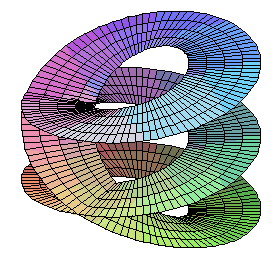The Riemann surface of the complex cube root function. Prerequisites: Mathematics 206H, 310H. Meetings: Three hours weekly. Instructor: Not offered

Math. 411 Introduction to mathematical modelling Fall-Winter 2004-2005

Differential equations, ordinary and partial.

Math.-Comp. Sci. 415H Mathematical Logic Fall 2004
 An introduction to the syntax and semantics of propositional and first-order logics through the Soundness, Completeness and Compactness Theorems. Prerequisite: Mathematics-Computer Science 260 or Mathematics 330 or permission of the instructor. Meetings: Two hours weekly. Class timetable Instructor:   Stefan Bilaniuk For more information, please see the MATH 415H home page.

Math.-Comp. Sci. 416H Computability Not offered in 2004-2005

An introduction to computability via Turing machines and recursive functions, followed either by applications to the Incompleteness Theorem or by an introduction to complexity theory.

• Prerequisite: Computer Science 305H or Mathematics 330 or Mathematics-Computer Science 415H or permission of the instructor.
• Instructor: Not offered

Math. 426H Geometry III: Topics in geometry Not offered in 2004-2005
• Prerequisite: Mathematics 225 or 226H or 326H.
• Meetings: To be arranged
• Instructor: Not offered

Math. 431H Algebra IV: Galois theory Fall 2004 (reading course)

Extension fields and Galois groups.

• Prerequisite: Mathematics 330.
• Meetings: To be arranged.
• Instructor:   David Poole

Math. 432H Algebra V: Topics in algebra Not offered in 2004-2005
• Prerequisite: Mathematics 330.
• Meetings: To be arranged
• Instructor: Not offered

Math. 436H Topology II: General topology Not offered in 2004-2005
• Prerequisite: Mathematics 310H
• Meetings: To be arranged
• Instructor: Not offered

Math. 437H Topology III: Topics in topology Not offered in 2004-2005
• Prerequisite: Mathematics 310H.
• Meetings: To be arranged
• Instructor: Not offered

Math. 451H Sampling theory Not offered in 2004-2005
• Prerequisites: Mathematics 355.
• Meetings: To be arranged
• Instructor: Not offered
• For more information, please see the Statistics Courses Page.

Math. 452H Theory of inference Not offered in 2004-2005
• Prerequisite: Mathematics 355.
• Meetings: To be arranged
• Instructor: Not offered
• For more information, please see the Statistics Courses Page.

Math. 460 Combinatorics and graph theory Not offered in 2004-2005
• Prerequisite: Mathematics-Computer Science 260 or permission ofthe instructor.
• Recommended: Mathematics 330.
• Meetings: Three hours weekly.
• Instructor: Not offered

Math. 470 Dynamical systems, chaos and fractals Fall-Winter 2004-2005
 Prerequisites: Mathematics 206H and 235H. Recommended: Mathematics 306H, 310H. Meetings: Three hours weekly. Class timetable Instructor:   Reem Yassawi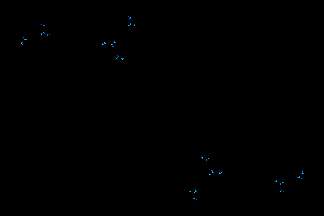The Julia set for $f\left(z\right) = z2+ c$. As $c$ moves, the Julia set changes.

Math. 490 Reading-seminar course (Full) Fall and Winter (reading course)
Details may be obtained by consulting the Department of Mathematics.
Math. 491H Perspectives in Mathematics Winter 2005

A survey of current research areas in mathematics, with two-week introductions to a variety of topics.

• Prerequisites: Mathematics 200, Math 235, and one 300-level mathematics course.
• Instructor: Several faculty members will participate

Math. 491H Reading-seminar course (Half) Fall or Winter (reading course)
Details may be obtained by consulting the Department of Mathematics.
Math. 495 Special Topics Fall-Winter 2004-2005 (reading course)
• Prerequisite: Permission of the instructor.
• Meetings: To be arranged.
• Instructor: